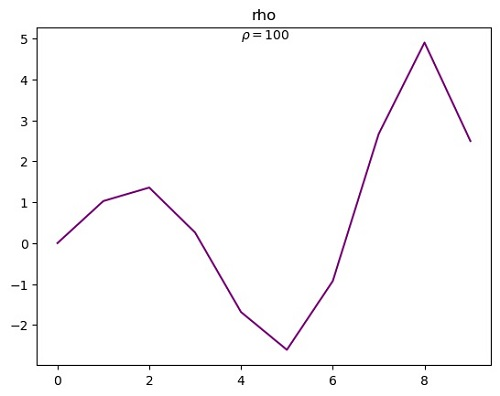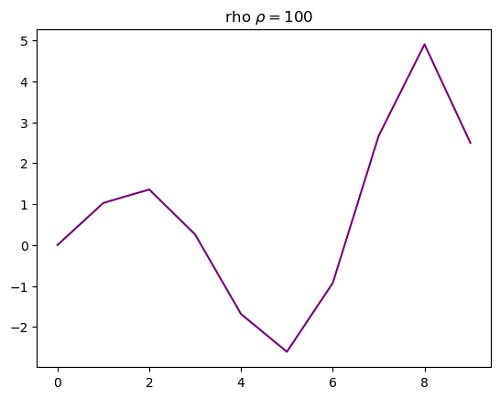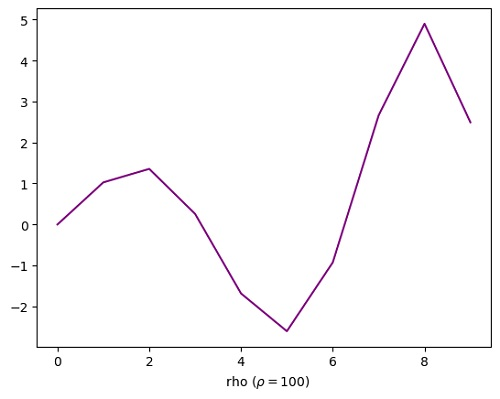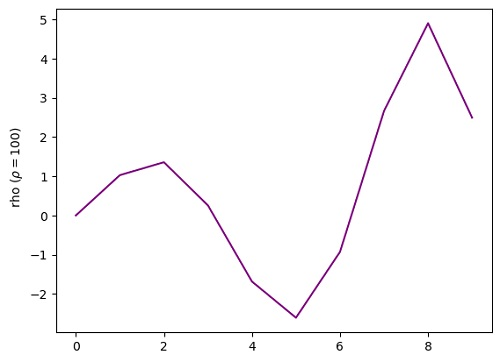# Python | Adding rho in Plot Label

Adding rho in Plot Label: Here, we are going to learn how to add rho in Python plot label?
Submitted by Anuj Singh, on July 23, 2020

⍴ (rho) is very often used greek mathematical letters. They are mostly used in physics, chemistry, fluid dynamics, and other mathematical expressions when there is topic related change (for example energy change, fluid density) and therefore, they have registered importance in languages.

Therefore, matplotlib has defined a command for usage.

plt.text(3, 0.4, r'$\rho=100$')plt.title(‘rho 'r'$\rho=100$')
#Adding ⍴ in title of the figureplt.xlabel('rho ('r'$\rho=100)$')
#Adding ⍴ in x axis label of the figureplt.ylabel('rho ('r'$\rho=100)$')
#Adding ⍴ in y axis label of the figure## Python code for adding rho in plot label

import numpy as np
import matplotlib.pyplot as plt

x = np.arange(10)
y = np.sin(x)*np.exp(x/5)

#  rho
#In text
plt.figure()
plt.plot(x,y, color=  'purple')
plt.title('rho')
plt.text(4, 5, r'$\rho=100$')

plt.show()

#In title
plt.figure()
plt.plot(x,y, color=  'purple')
plt.title('  rho 'r'$\rho=100$')

plt.show()

#In x-axis label
plt.figure()
plt.plot(x,y, color=  'purple')
plt.xlabel('  rho ('r'$\rho=100)$')

plt.show()

#In y-axis label
plt.figure()
plt.plot(x,y, color=  'purple')
plt.ylabel('  rho ('r'$\rho=100)$')

plt.show()


Output:

Output is as figure


Languages: » C » C++ » C++ STL » Java » Data Structure » C#.Net » Android » Kotlin » SQL
Web Technologies: » PHP » Python » JavaScript » CSS » Ajax » Node.js » Web programming/HTML
Solved programs: » C » C++ » DS » Java » C#
Aptitude que. & ans.: » C » C++ » Java » DBMS
Interview que. & ans.: » C » Embedded C » Java » SEO » HR
CS Subjects: » CS Basics » O.S. » Networks » DBMS » Embedded Systems » Cloud Computing
» Machine learning » CS Organizations » Linux » DOS
More: » Articles » Puzzles » News/Updates